Examples

Chapter 7 Class 8 Comparing Quantities
Serial order wise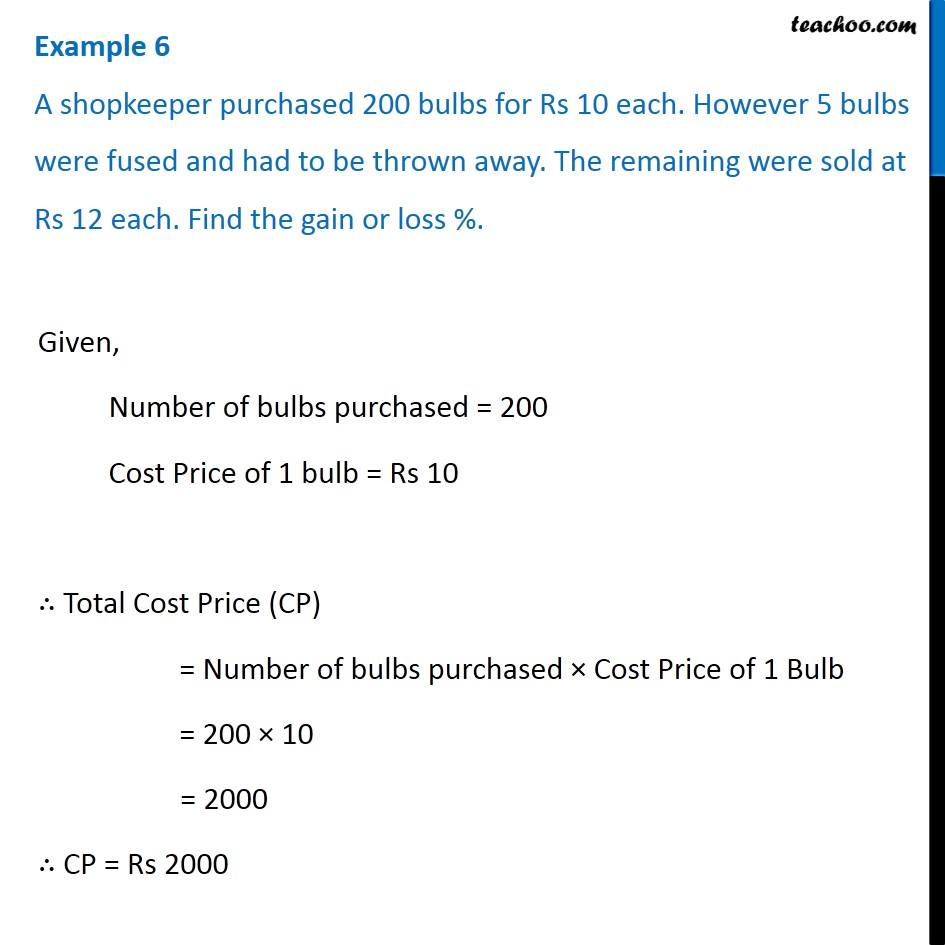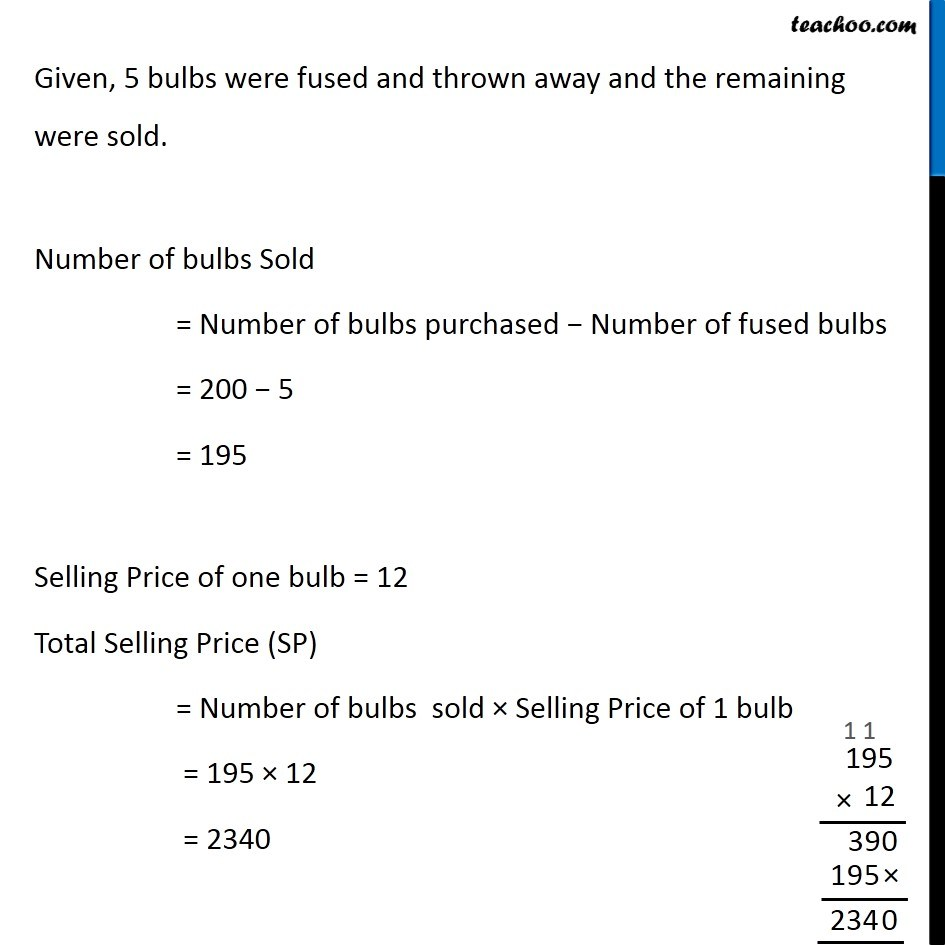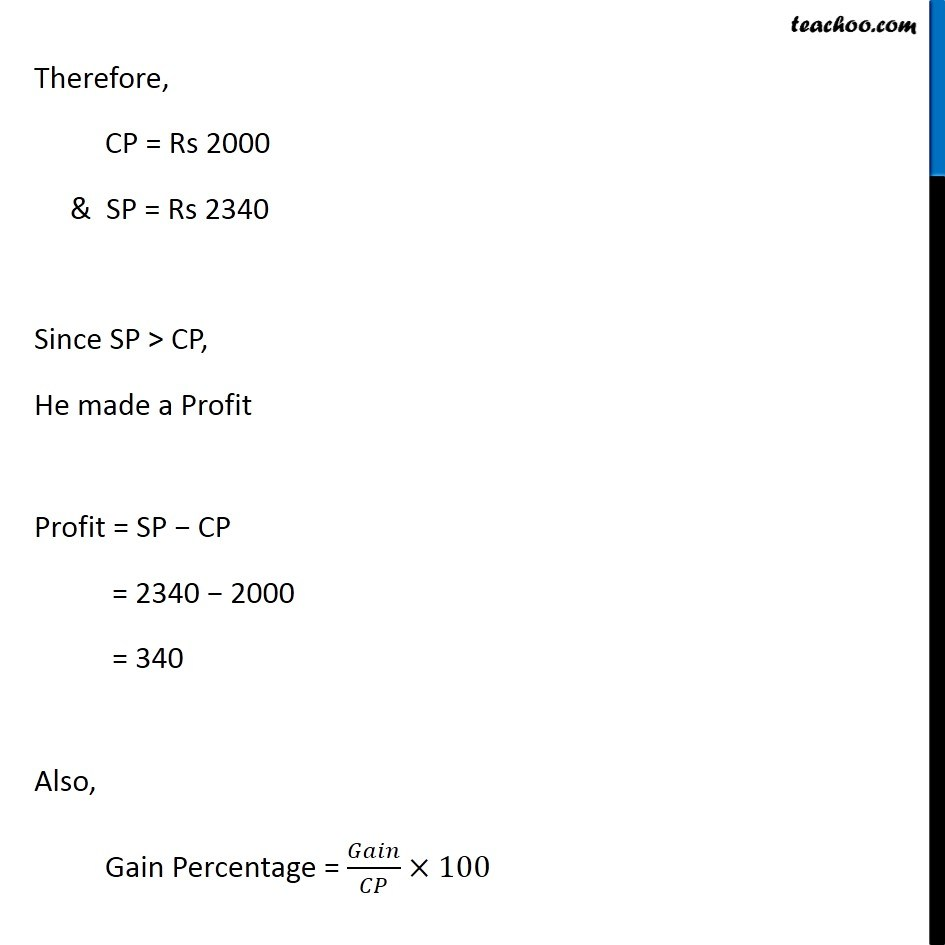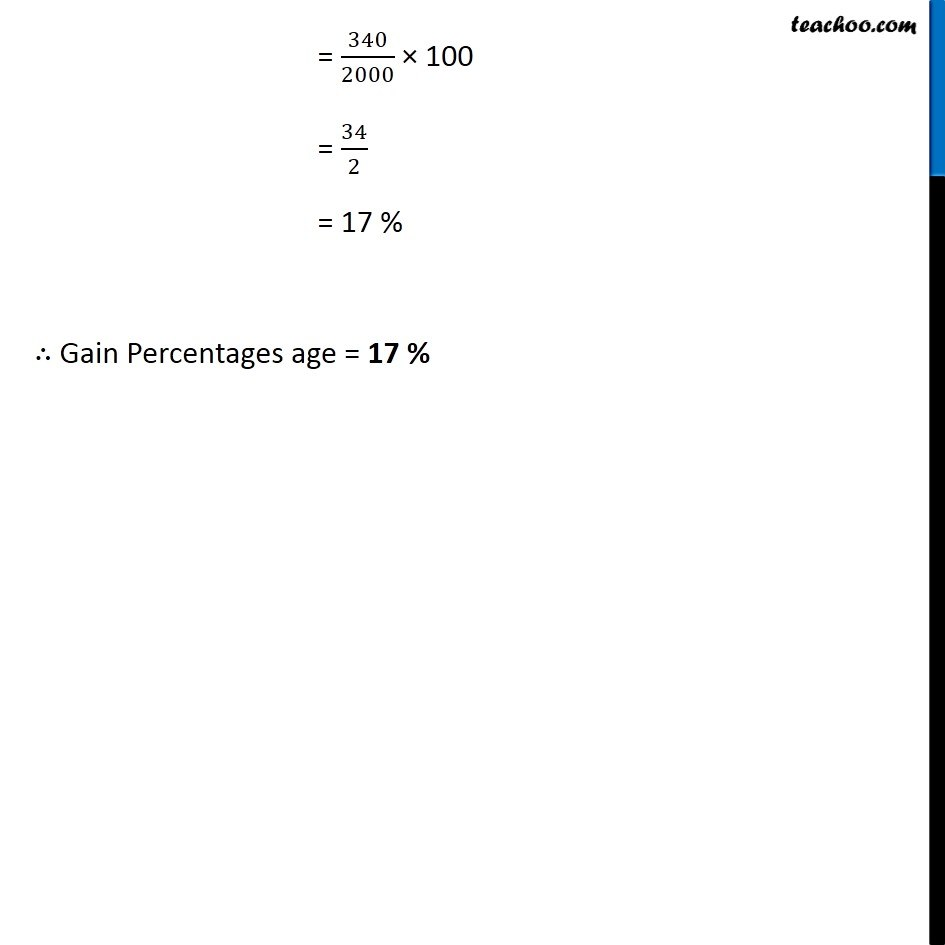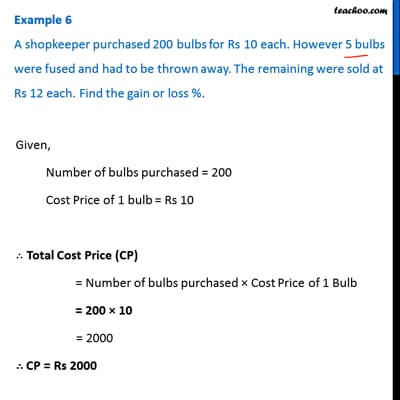This video is only available for Teachoo black users

Learn in your speed, with individual attention - Teachoo Maths 1-on-1 Class

### Transcript

Question 3 A shopkeeper purchased 200 bulbs for Rs 10 each. However 5 bulbs were fused and had to be thrown away. The remaining were sold at Rs 12 each. Find the gain or loss %. Given, Number of bulbs purchased = 200 Cost Price of 1 bulb = Rs 10 ∴ Total Cost Price (CP) = Number of bulbs purchased × Cost Price of 1 Bulb = 200 × 10 = 2000 ∴ CP = Rs 2000 Given, 5 bulbs were fused and thrown away and the remaining were sold. Number of bulbs Sold = Number of bulbs purchased − Number of fused bulbs = 200 − 5 = 195 Selling Price of one bulb = 12 Total Selling Price (SP) = Number of bulbs sold × Selling Price of 1 bulb = 195 × 12 = 2340 Therefore, CP = Rs 2000 & SP = Rs 2340 Since SP > CP, He made a Profit Profit = SP − CP = 2340 − 2000 = 340 Also, Gain Percentage = 𝐺𝑎𝑖𝑛/𝐶𝑃×100 = 340/2000 × 100 = 34/2 = 17 % ∴ Gain Percentages age = 17 %